# "Either/Or" Probability for Overlapping Events in a Venn Diagram

Author: Sophia Tutorial
##### Description:

Calculate an "either/or" probability for overlapping events in a Venn diagram.

(more)

Sophia’s self-paced online courses are a great way to save time and money as you earn credits eligible for transfer to many different colleges and universities.*

No credit card required

38 Sophia partners guarantee credit transfer.

299 Institutions have accepted or given pre-approval for credit transfer.

* The American Council on Education's College Credit Recommendation Service (ACE Credit®) has evaluated and recommended college credit for 33 of Sophia’s online courses. Many different colleges and universities consider ACE CREDIT recommendations in determining the applicability to their course and degree programs.

Tutorial
what's covered
In this tutorial, you're going to learn about “or” probability for overlapping events. Our discussion breaks down as follows:

1. Either/Or Probability for Overlapping Events
2. Either/Or Probability for Overlapping Events With Two-Way Tables
3. General Addition Rule for Non-Overlapping Events

## 1. Either/Or Probability for Overlapping Events

Either/or probability for overlapping events refers to the probability of either A, or B, or both, occurring.

EXAMPLE

Consider the roulette wheel below.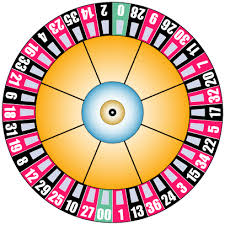What's the probability of getting an even number on a roulette wheel? This is not an “or” probability. We're simply finding the probability of spinning an even number. Looking just in the even circle, 18 numbers are even on the roulette wheel, out of 38 sectors. Zero and double zero don't count as even.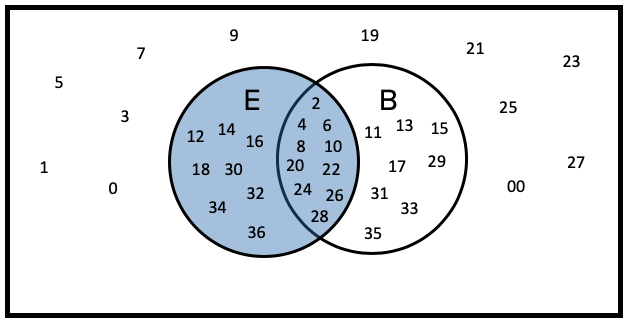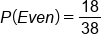What is the probability of black on the roulette wheel? It's the same idea. Look in the circle that represents black numbers, it's also 18 out of 38, coincidentally the same number.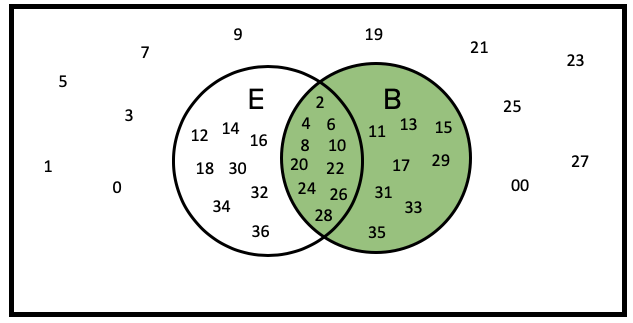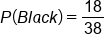What's the probability of even or black? Count up the numbers that are in either even or black. You get 26 out of the 38.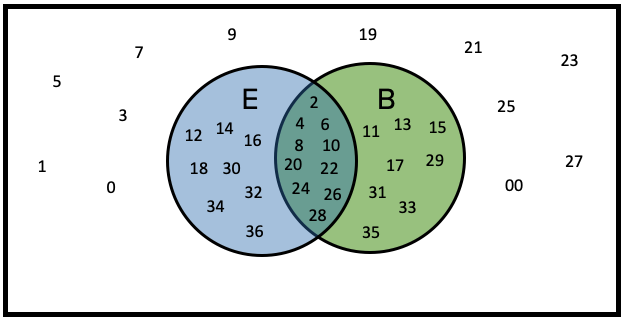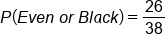Now the question is, why is the probability of E or B not equal to the probability of E plus probability of B?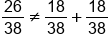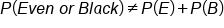When you add the probability of an even number plus the probability of a black number, you should get 36/38, but you don't--you get 26/38. The reason for the miscount is because some of the numbers were counted twice. Some were counted as even, and some were counted again as being black, whereas we should only have counted them once.

Which values were double counted? It was the numbers that were even and black. You can add these together, except you'll subtract out the things that got double counted so they are only counted once. The double counts were the numbers that were both even and black, represented in the overlap of the Venn diagram.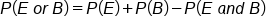This represents the either/or probability for overlapping events: the probability of E or B is equal to the probability of E plus the probability of B, minus the probability of E and B.
Let's illustrate this concept by boxing the probability of all even numbers and circling the probability of B numbers.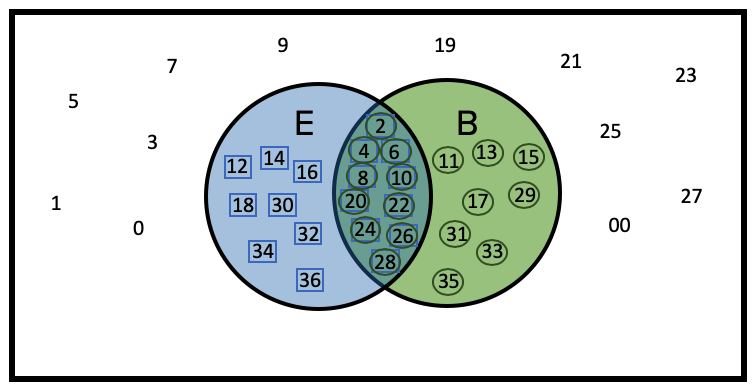Notice how the numbers in the middle got both circled and boxed. You only want to count them once. But when we include the numbers with the E’s and also with the B’s, they were counted twice. Remove one of those markings to only count everything once.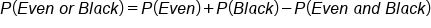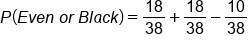formula
Either/Or Probability for Overlapping Events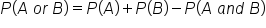term to know

Either/Or Probability for Overlapping Events
The probability that either of two events occurs is equal to the sum of the probabilities of the two events, minus the joint probability of the two events happening together. Also known as the "General Addition Rule".

## 2. Either/Or Probability for Overlapping Events With Two-Way Tables

Let's look at another example using a two-way table, shown below. Students in the middle school were asked about their dominant hand. Some are right-handed sixth graders, left-handed sixth graders, etc.

 Dominant Hand Grade Right Left Ambidextrous 6th 99 9 2 110 7th 90 31 0 121 8th 93 11 3 107 282 51 5 338

What's the probability that a student is either in eighth grade or left-handed?

The probability of eighth grade or left-handed is equal to the probability of being an eighth grader plus the probability of being a left-handed student minus the probability of both.Why minus the probability of both? Because you counted the left-handed eighth graders in the eighth-grade row and in the left-hand column. We double counted those 11 students, and you only want to count them once. Add and subtract those probabilities as shown below, and you end up with 147/338.

The other way to approach this is by simply adding up the cells that are either left-handed or eighth grade.

 Dominant Hand Grade Right Left Ambidextrous 6th 99 9 2 110 7th 90 31 0 121 8th 93 11 3 107 282 51 5 338

## 3. General Addition Rule for Non-Overlapping Events

It’s important to note, this formula also works for non-overlapping events. Since mutually exclusive events, A and B, can't happen at the same time, the probability that both A and B occur is zero. It's impossible for A and B to both happen.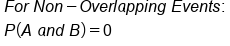Therefore, when we factor that into our formula, we end up with the probability of A plus the probability of B minus 0. When it simplifies down, you may recognize this. This was the special addition rule for non-overlapping events. What we see, then, is that the special addition rule for non-overlapping events is a special case of the general addition rule.summary
Either/or probability for overlapping events is calculated by adding the probabilities of the two events, then subtracting their joint probability. The reason you subtract out the joint probability is that it is counted in both of their individual probabilities. You don't want to count it twice; you only want it counted once. With mutually exclusive (non-overlapping) events, the joint probability is zero, and it simplifies down to the special addition rule, which is a special case of the general addition rule.

Good luck!

Source: Adapted from Sophia tutorial by Jonathan Osters.

Terms to Know
Either/Or Probability for Overlapping Events

The probability that either of two events occurs is equal to the sum of the probabilities of the two events, minus the joint probability of the two events happening together.  Also known as the "General Addition Rule".

Formulas to Know
Either/Or Probability for Overlapping EventsRating